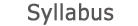2019 - 2020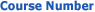0509-2801-01
Intr. to Probability and Statistics
FACULTY OF ENGINEERING | DEPARTMENTS OF ENGINEERING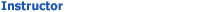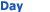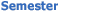Efrat Naamati Engineering Studies - Classrooms 102 Wed 1300-1600 Sem  1
University credit hours:  4.0

Course description
Credit points: 3.5
Prerequisites: Differential and Integral Calculus.
Combinatorics, Sample space and events, probability function, conditional probability and Bayes theorem, Random Variables, distribution and conditional distribution, expectation and variance. Markov and Chebyshev inequalities, moments, characteristic function, distributions: Binomial. Geometric, Poison, Exponential. Gaussian, Functions of random variables, Bivariate distributions: joint and marginal distributions, functions of two random variables, joint moments, joint characteristic function, conditional distribution and conditional expectation, expectation theorem. Random vector, random series, weak law of large numbers, central limit theorem, parameters estimation, confidence intervals for the expected value, Bayesian approach, maximum likelihood, Rau Cramer inequality, tests of hypothesis.

accessibility declaration